You are currently offline. Some features of the site may not work correctly.

# Quotient graph

In graph theory, a quotient graph Q of a graph G is a graph whose vertices are blocks of a partition of the vertices of G and where block B is… Expand
Wikipedia

## Papers overview

Semantic Scholar uses AI to extract papers important to this topic.
2019
2019
• IEEE 60th Annual Symposium on Foundations of…
• 2019
• Corpus ID: 104292168
We show that planar graphs have bounded queue-number, thus proving a conjecture of Heath, Leighton and Rosenberg from 1992. The… Expand
•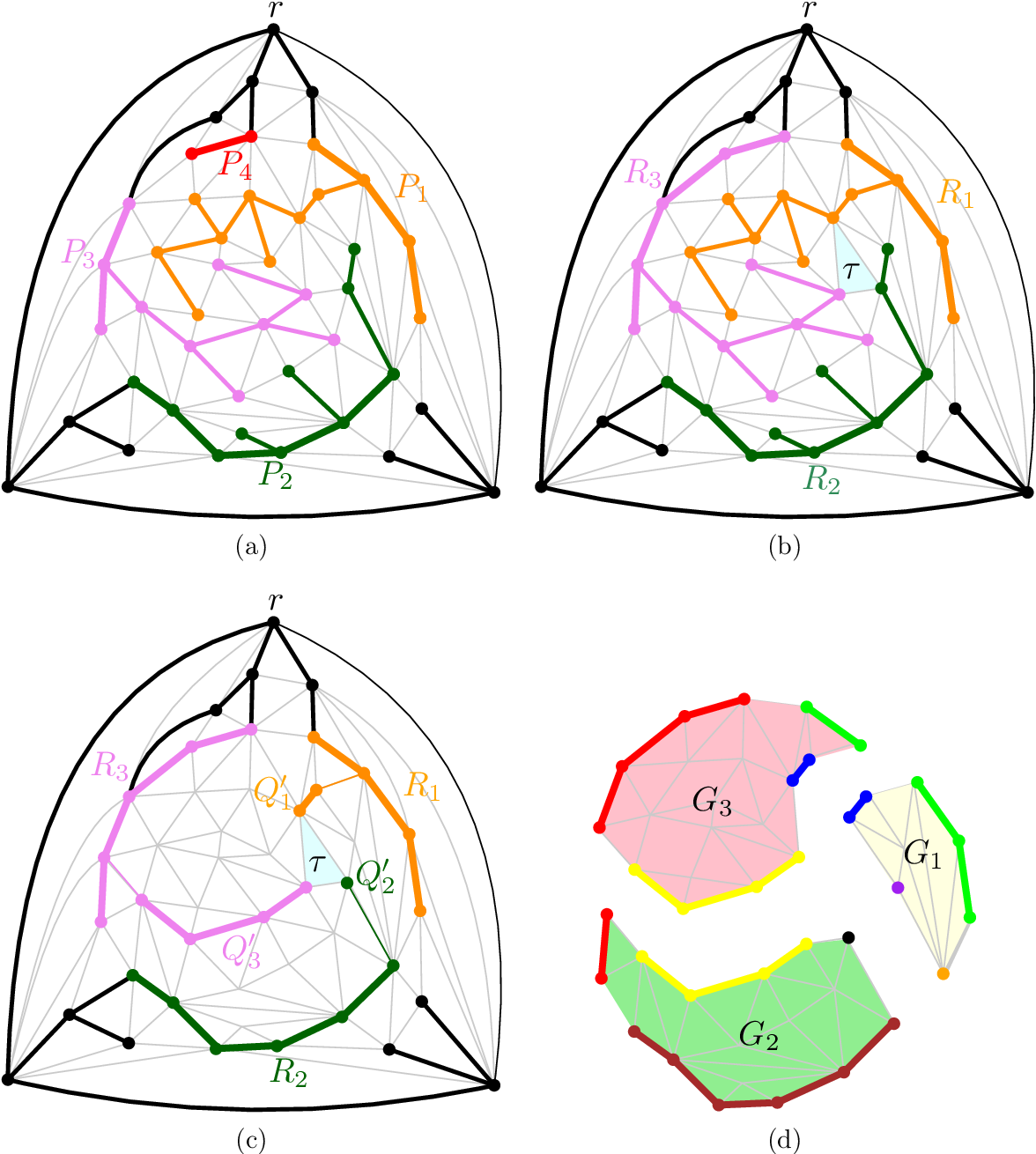•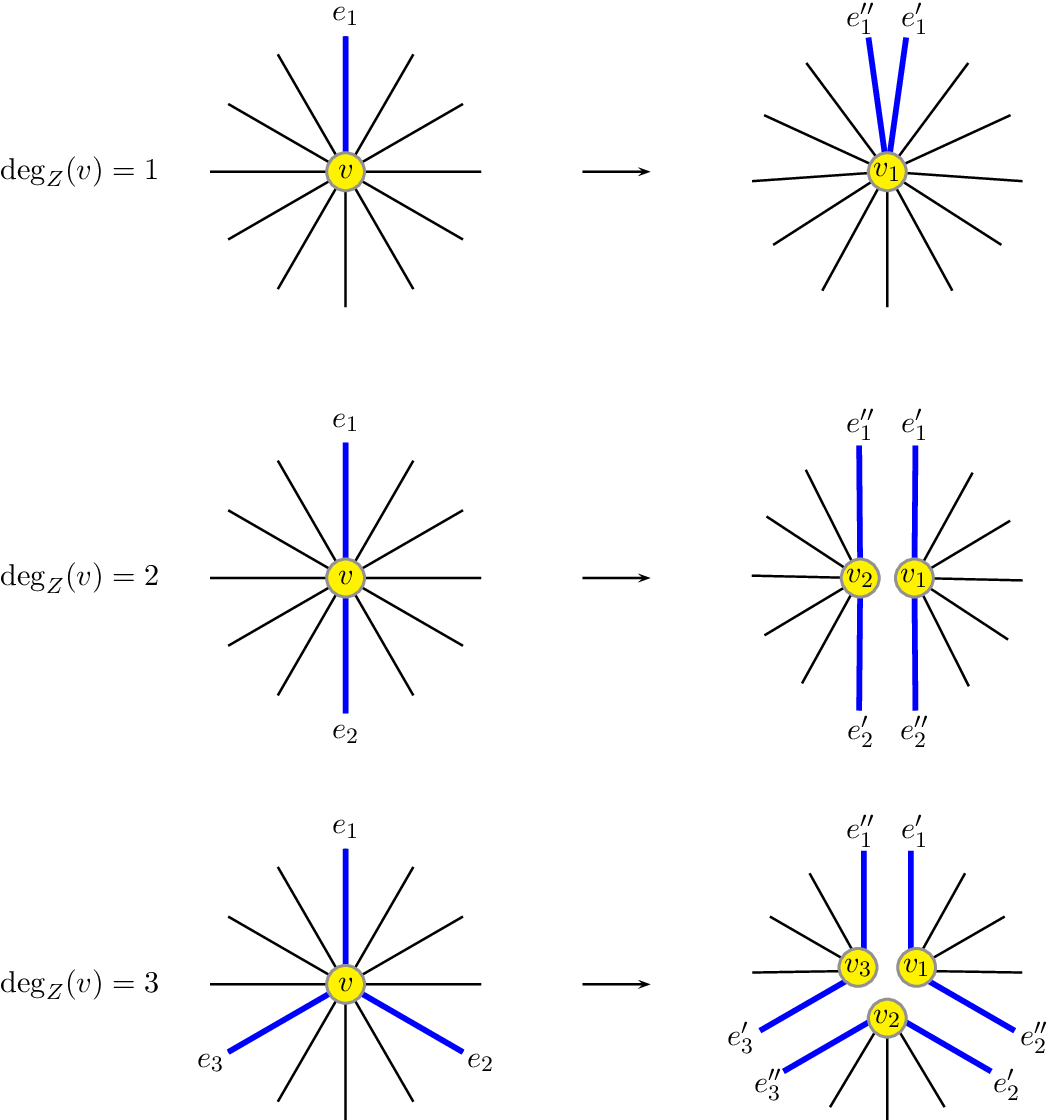Is this relevant?
2008
2008
The notion of strongly quotient graph was introduced by Adiga et al. (3). Here, we show that some well known families of graphs… Expand
•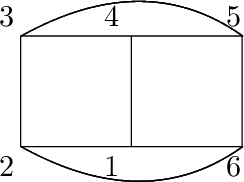•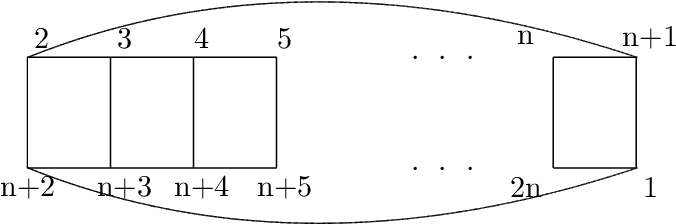••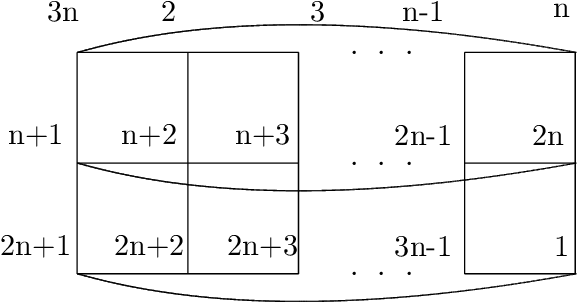•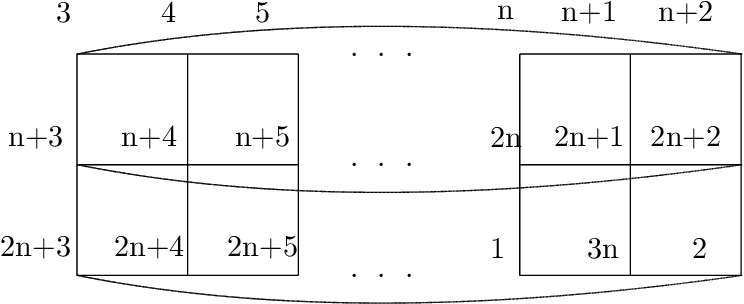Is this relevant?
2007
2007
• J. Discrete Algorithms
• 2007
• Corpus ID: 1774102
In this paper we propose a dynamic programming algorithm to evaluate local similarity between ordered quotiented trees using a… Expand
•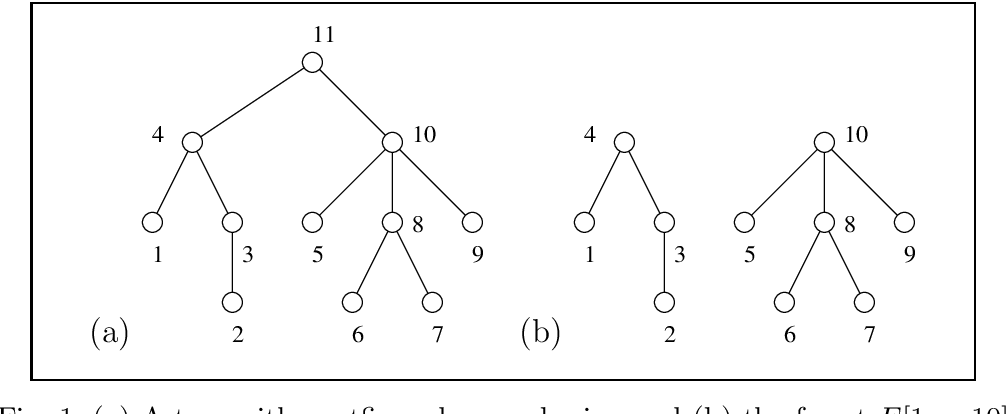•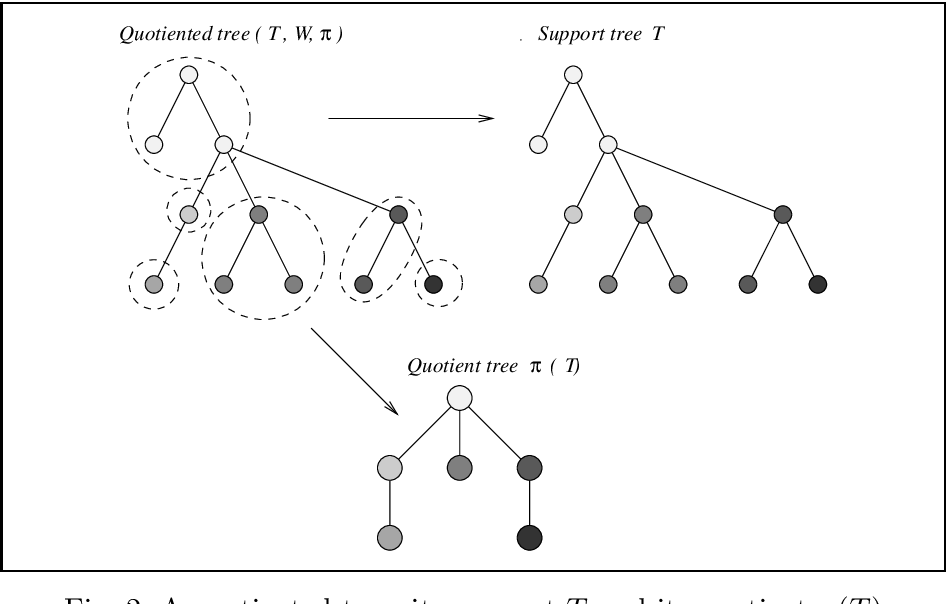•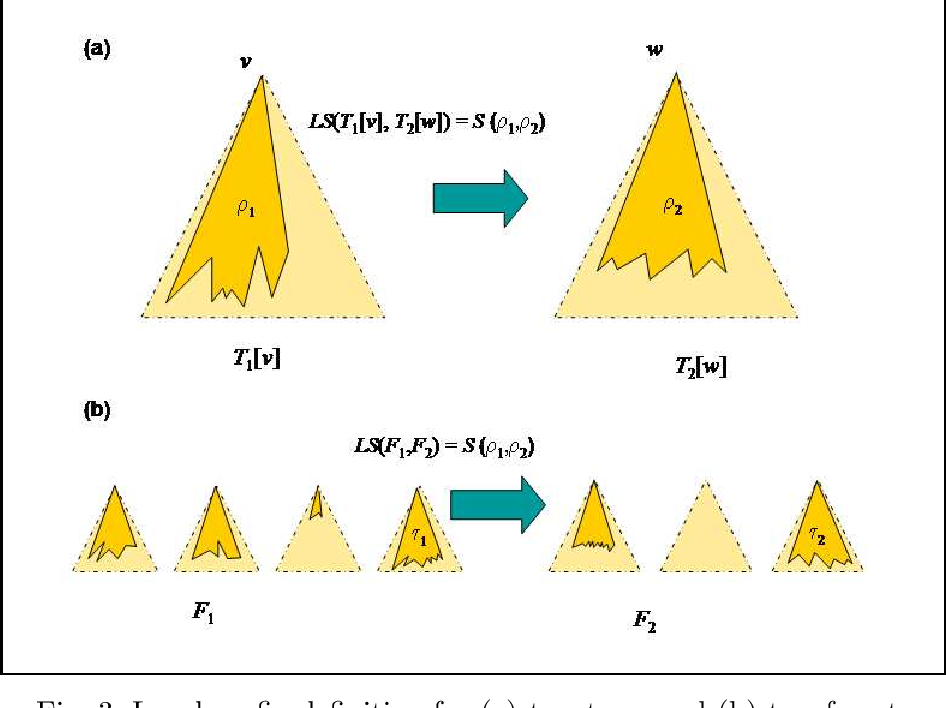•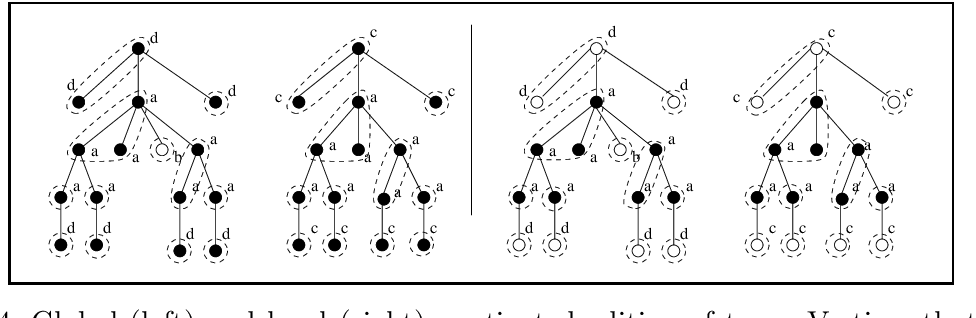•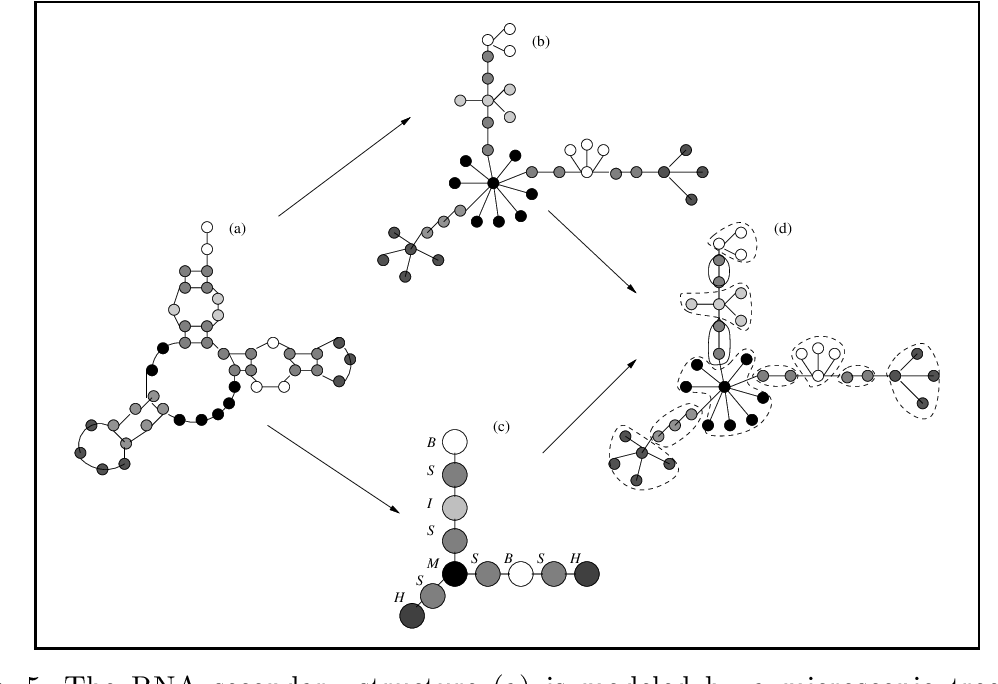Is this relevant?
2007
2007
• SIAM J. Discret. Math.
• 2007
• Corpus ID: 6248140
A graph \$G=(V,E)\$ is a chordal probe graph if its vertices can be partitioned into two sets, \$P\$ (probes) and \$N\$ (non-probes… Expand
•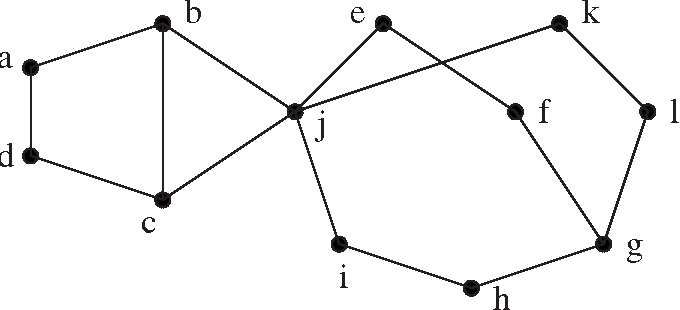•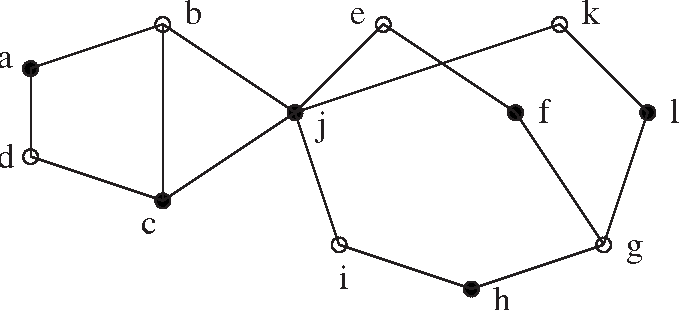•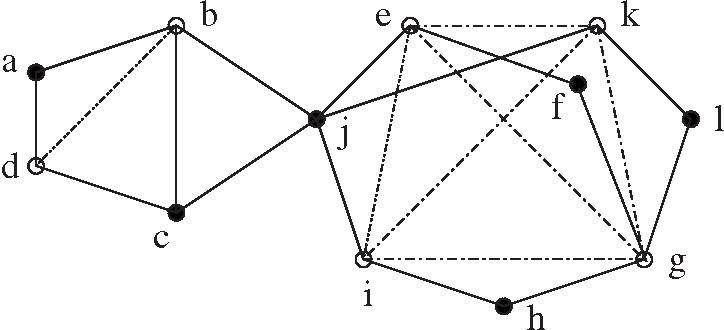•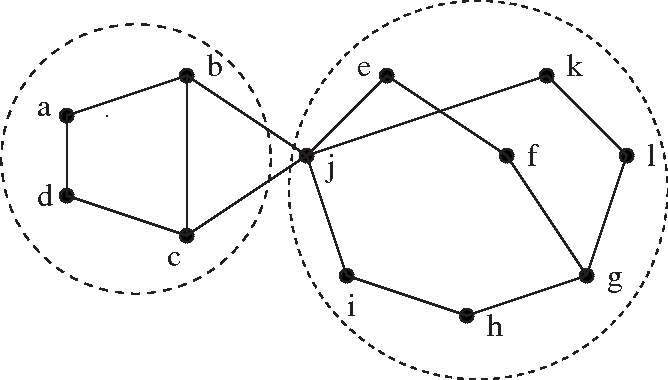•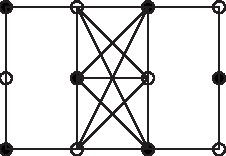Is this relevant?
2005
2005
• J. Comb. Theory, Ser. B
• 2005
• Corpus ID: 29793155
This paper forms part of a study of 2-arc transitivity for finite imprimitive symmetric graphs, namely for graphs Γ admitting an… Expand
Is this relevant?
2000
2000
• 2000
• Corpus ID: 3167081
A predictive scanning input system provides predictive selection of individual indicators corresponding to keys on the keyboard… Expand
Is this relevant?
2000
2000
• 2000
• Corpus ID: 11781652
Let Γ be a finite G-symmetric graph whose vertex set admits a non-trivial Ginvariant partition B with block size v. A framework… Expand
•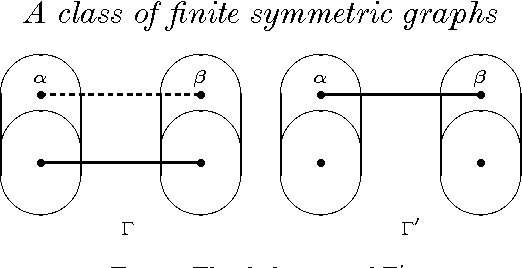•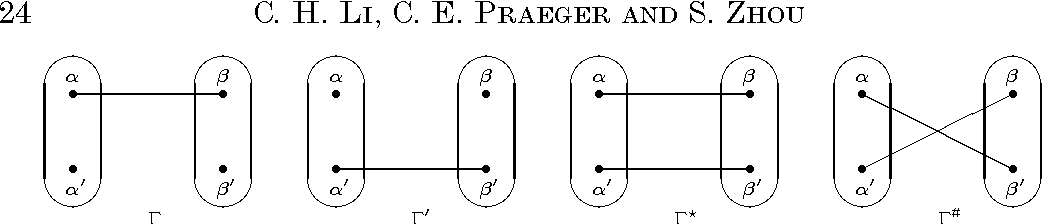•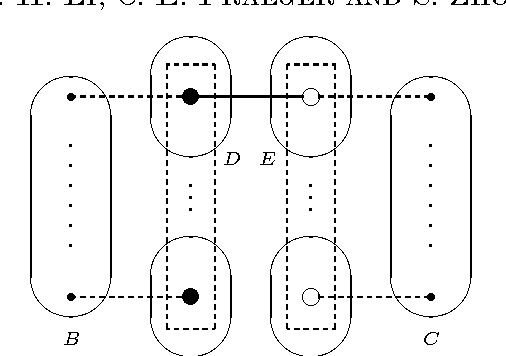Is this relevant?
Highly Cited
1996
Highly Cited
1996
• SIAM J. Matrix Anal. Appl.
• 1996
• Corpus ID: 6545163
An approximate minimum degree (AMD) ordering algorithm for preordering a symmetric sparse matrix prior to numerical factorization… Expand
Is this relevant?
1989
1989
Abstract Using a standard notion of a quotient graph of certain vertex-transitive graphs, methods for lifting a Hamilton cycle in… Expand
Is this relevant?
1986
1986
The partitioning of the vertices of an undirected graph, in a way that makes its quotient graph a tree, mirrors a way of… Expand
Is this relevant?